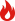﻿ 制图机械报价及图片大全_中国教育装备采购网大家都在搜
排序
默认发布时间
第 1 / 页
共 1 条
• 品　　牌：峰启
产品型号：FQ-CCM
拆装减速器技术参数双级园柱齿轮减速器(展开式)535*330*294M=3Z1=16Z2=47Z3=27Z4=68=20度=15度I=7.398a1=99a2=147H=14... 【详细信息】【有28个相关】